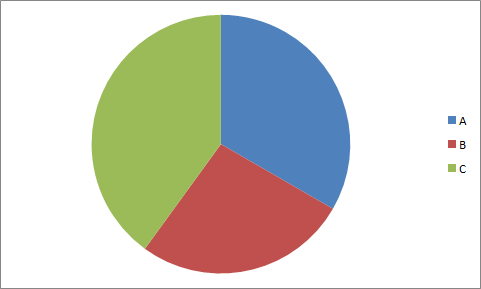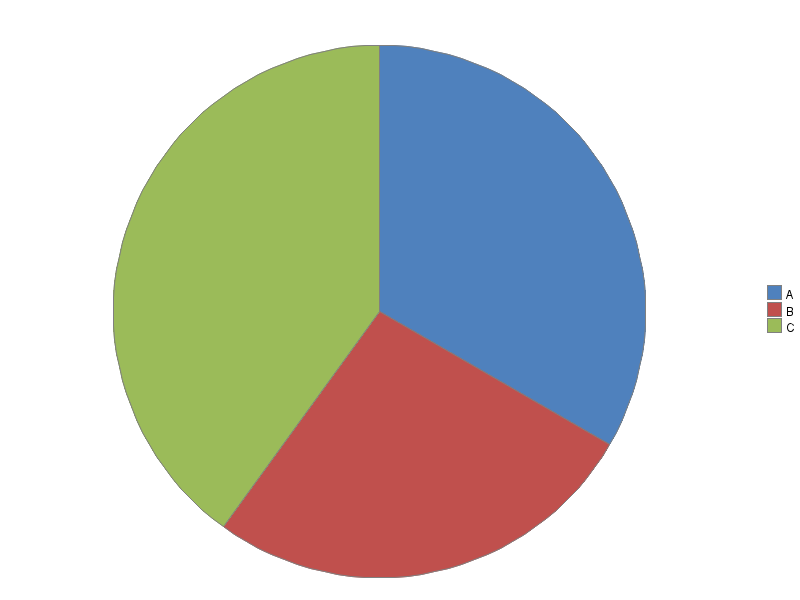## Pies with Horizontal Data

I would like to create a pie chart with data that exist in the following format. How can SAS do it? I have looked into the GChart procedure, but it looks like my data need to be in the format below the graph to be able to work... Any ideas?

Data that I have:

 A B C 5 4 6

Chart that I need:Data that Proc GChart needs to produce a pie:

 A A A A A B B B B C C C C C C
1 ACCEPTED SOLUTION

Accepted Solutions

## Re: Pies with Horizontal Data

Here's how to create a pie chart like that with Gchart ...

data my_data;
input slice_var \$1 quantity;
datalines;
A 5
B 4
C 6
;
run;

legend1 label=none across=1 position=(right middle) shape=bar(.15in,.15in);

pattern1 v=s c=cx4f81bd;
pattern2 v=s c=cxc0504d;
pattern3 v=s c=cx9bbb59;

proc gchart data=my_data;
pie slice_var / type=sum sumvar=quantity
angle=90 clockwise;
run;3 REPLIES 3

## Re: Pies with Horizontal Data

Here's how to create a pie chart like that with Gchart ...

data my_data;
input slice_var \$1 quantity;
datalines;
A 5
B 4
C 6
;
run;

legend1 label=none across=1 position=(right middle) shape=bar(.15in,.15in);

pattern1 v=s c=cx4f81bd;
pattern2 v=s c=cxc0504d;
pattern3 v=s c=cx9bbb59;

proc gchart data=my_data;
pie slice_var / type=sum sumvar=quantity
angle=90 clockwise;
run;## Re: Pies with Horizontal Data

You might also find my Pie Chart tutorial useful!http://blogs.sas.com/content/sastraining/2013/09/17/a-beginners-tutorial-on-pie-charts/

## Re: Pies with Horizontal Data

Awesome!

Thank youDiscussion stats
• 3 replies
• 825 views
• 3 likes
• 2 in conversation